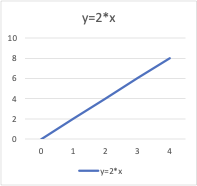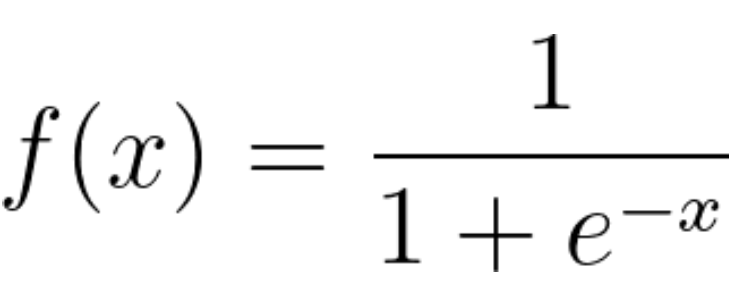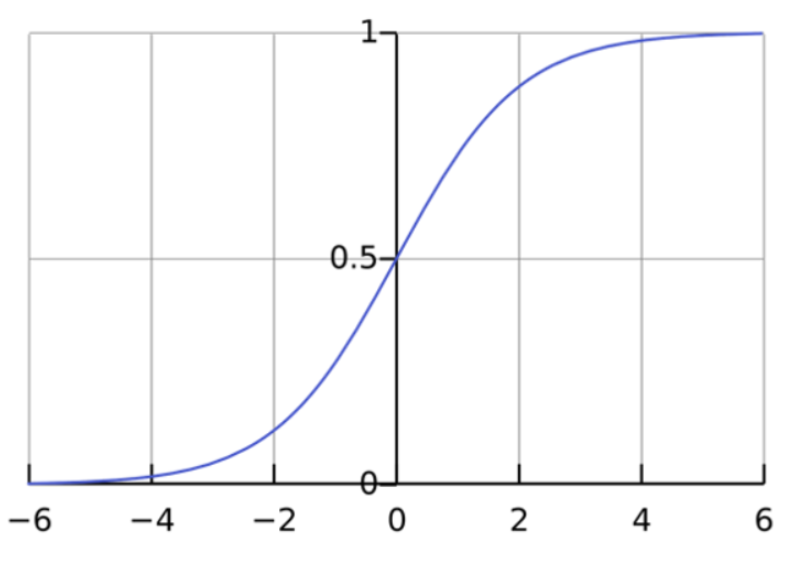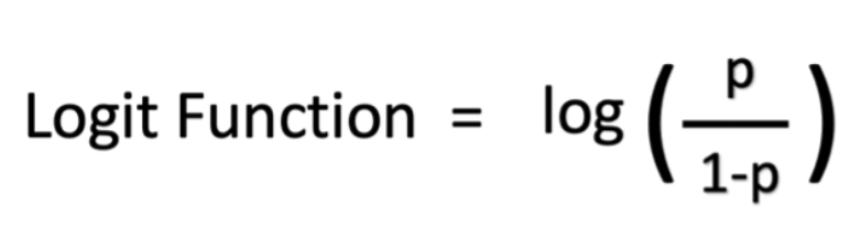# What is Logistic Regression?

## What is logistic regression?

Logistic regression is a data analysis technique that uses mathematics to find the relationships between two data factors. It then uses this relationship to predict the value of one of those factors based on the other. The prediction usually has a finite number of outcomes, like yes or no.

For example, let’s say you want to guess if your website visitor will click the checkout button in their shopping cart or not. Logistic regression analysis looks at past visitor behavior, such as time spent on the website and the number of items in the cart. It determines that, in the past, if visitors spent more than five minutes on the site and added more than three items to the cart, they clicked the checkout button. Using this information, the logistic regression function can then predict the behavior of a new website visitor.

## Why is logistic regression important?

Logistic regression is an important technique in the field of artificial intelligence and machine learning (AI/ML). ML models are software programs that you can train to perform complex data processing tasks without human intervention. ML models built using logistic regression help organizations gain actionable insights from their business data. They can use these insights for predictive analysis to reduce operational costs, increase efficiency, and scale faster. For example, businesses can uncover patterns that improve employee retention or lead to more profitable product design.

Below, we list some benefits of using logistic regression over other ML techniques.

### Simplicity

Logistic regression models are mathematically less complex than other ML methods. Therefore, you can implement them even if no one on your team has in-depth ML expertise.

### Speed

Logistic regression models can process large volumes of data at high speed because they require less computational capacity, such as memory and processing power. This makes them ideal for organizations that are starting with ML projects to gain some quick wins.

### Flexibility

You can use logistic regression to find answers to questions that have two or more finite outcomes. You can also use it to preprocess data. For example, you can sort data with a large range of values, such as bank transactions, into a smaller, finite range of values by using logistic regression. You can then process this smaller data set by using other ML techniques for more accurate analysis.

### Visibility

Logistic regression analysis gives developers greater visibility into internal software processes than do other data analysis techniques. Troubleshooting and error correction are also easier because the calculations are less complex.

## What are the applications of logistic regression?

Logistic regression has several real-world applications in many different industries.

### Manufacturing

Manufacturing companies use logistic regression analysis to estimate the probability of part failure in machinery. They then plan maintenance schedules based on this estimate to minimize future failures.

### Healthcare

Medical researchers plan preventive care and treatment by predicting the likelihood of disease in patients. They use logistic regression models to compare the impact of family history or genes on diseases.

### Finance

Financial companies have to analyze financial transactions for fraud and assess loan applications and insurance applications for risk. These problems are suitable for a logistic regression model because they have discrete outcomes, like high risk or low risk and fraudulent or not fraudulent.

### Marketing

Online advertising tools use the logistic regression model to predict if users will click on an advertisement. As a result, marketers can analyze user responses to different words and images and create high-performing advertisements with which customers will engage.

## How does regression analysis work?

Logistic regression is one of several different regression analysis techniques that data scientists commonly use in machine learning (ML). To understand logistic regression, we must first understand basic regression analysis. Below, we use an example of linear regression analysis to demonstrate how regression analysis works.

### Identify the question

Any data analysis begins with a business question. For logistic regression, you should frame the question to get particular outcomes:

• Do rainy days impact our monthly sales? (yes or no)
• What type of credit card activity is the customer performing? (authorized, fraudulent, or potentially fraudulent)

### Collect historical data

After identifying the question, you need to identify the data factors that are involved. You will then collect past data for all factors. For example, to answer the first question shown above, you could collect the number of rainy days and your monthly sales data for each month in the past three years.

### Train the regression analysis model

You will process the historical data using regression software. The software will process the different data points and connect them mathematically by using equations. For example, if the number of rainy days for three months are 3, 5, and 8 and the number of sales in those months are 8, 12, and 18, the regression algorithm will connect the factors with the equation:

Number of Sales = 2*(Number of Rainy Days) + 2

### Make predictions for unknown values

For unknown values, the software uses the equation to make a prediction. If you know that it will rain for six days in July, the software will estimate July’s sale value as 14.

## How does the logistic regression model work?

To understand the logistic regression model, let’s first understand equations and variables.

### Equations

In mathematics, equations give the relationship between two variables: x and y. You can use these equations, or functions, to plot a graph along the x-axis and y-axis by putting in different values of x and y. For instance, if you plot the graph for the function y = 2*x, you will get a straight line as shown below. Hence this function is also called a linear function.### Variables

In statistics, variables are the data factors or attributes whose values vary. For any analysis, certain variables are independent or explanatory variables. These attributes are the cause of an outcome. Other variables are dependent or response variables; their values depend on the independent variables. In general, logistic regression explores how independent variables affect one dependent variable by looking at historical data values of both variables.

In our example above, x is called the independent variable, predictor variable, or explanatory variable because it has a known value. Y is called the dependent variable, outcome variable, or response variable because its value is unknown.

### Logistic regression function

Logistic regression is a statistical model that uses the logistic function, or logit function, in mathematics as the equation between x and y. The logit function maps y as a sigmoid function of x.If you plot this logistic regression equation, you will get an S-curve as shown below.As you can see, the logit function returns only values between 0 and 1 for the dependent variable, irrespective of the values of the independent variable. This is how logistic regression estimates the value of the dependent variable. Logistic regression methods also model equations between multiple independent variables and one dependent variable.

### Logistic regression analysis with multiple independent variables

In many cases, multiple explanatory variables affect the value of the dependent variable. To model such input datasets, logistic regression formulas assume a linear relationship between the different independent variables. You can modify the sigmoid function and compute the final output variable as

y = f0 + β1x1 + β2x2+… βnxn)

The symbol β represents the regression coefficient. The logit model can reverse calculate these coefficient values when you give it a sufficiently large experimental dataset with known values of both dependent and independent variables.

### Log odds

The logit model can also determine the ratio of success to failure or log odds. For example, if you were playing poker with your friends and you won four matches out of 10, your odds of winning are four sixths, or four out of six, which is the ratio of your success to failure. The probability of winning, on the other hand, is four out of 10.

Mathematically, your odds in terms of probability are p/(1 - p), and your log odds are log (p/(1 - p)). You can represent the logistic function as log odds as shown below:## What are the types of logistic regression analysis?

There are three approaches to logistic regression analysis based on the outcomes of the dependent variable.

### Binary logistic regression

Binary logistic regression works well for binary classification problems that have only two possible outcomes. The dependent variable can have only two values, such as yes and no or 0 and 1.

Even though the logistic function calculates a range of values between 0 and 1, the binary regression model rounds the answer to the closest values. Generally, answers below 0.5 are rounded to 0, and answers above 0.5 are rounded to 1, so that the logistic function returns a binary outcome.

### Multinomial logistic regression

Multinomial regression can analyze problems that have several possible outcomes as long as the number of outcomes is finite. For example, it can predict if house prices will increase by 25%, 50%, 75%, or 100% based on population data, but it cannot predict the exact value of a house.

Multinomial logistic regression works by mapping outcome values to different values between 0 and 1. Since the logistic function can return a range of continuous data, like 0.1, 0.11, 0.12, and so on, multinomial regression also groups the output to the closest possible values.

### Ordinal logistic regression

Ordinal logistic regression, or the ordered logit model, is a special type of multinomial regression for problems in which numbers represent ranks rather than actual values. For example, you would use ordinal regression to predict the answer to a survey question that asks customers to rank your service as poor, fair, good, or excellent based on a numerical value, such as the number of items they purchase from you over the year.

## How does logistic regression compare to other ML techniques?

The two common data analysis techniques are linear regression analysis and deep learning.

### Linear regression analysis

As explained above, linear regression models the relationship between dependent and independent variables by using a linear combination. The linear regression equation is

y= β0X0 + β1X1 + β2X2+… βnXn+ ε, where β1 to βn and ε are regression coefficients.

#### Logistic regression vs. linear regression

Linear regression predicts a continuous dependent variable by using a given set of independent variables. A continuous variable can have a range of values, such as price or age. So linear regression can predict actual values of the dependent variable. It can answer questions like "What will the price of rice be after 10 years?"

Unlike linear regression, logistic regression is a classification algorithm. It cannot predict actual values for continuous data. It can answer questions like "Will the price of rice increase by 50% in 10 years?"

### Deep learning

Deep learning uses neural networks or software components that simulate the human brain to analyze information. Deep learning calculations are based on the mathematical concept of vectors.

#### Logistic regression vs. deep learning

Logistic regression is less complex and less compute intensive than deep learning. More importantly, deep learning calculations cannot be investigated or modified by developers, due to their complex, machine-driven nature. On the other hand, logistic regression calculations are transparent and easier to troubleshoot.

## How can you run logistic regression analysis on AWS?

You can run logistic regression on AWS by using Amazon SageMaker. SageMaker is a fully managed machine learning (ML) service with built-in algorithms for linear regression and logistic regression, among several other statistical software packages.

• Every data scientist can use SageMaker to prepare, build, train, and deploy logistic regression models quickly.
• SageMaker removes the heavy lifting from each step of the logistic regression process to make it easier to develop high-quality models.
• SageMaker provides all of the components you need for logistic regression in a single tool set so that you can get models to production faster, easier, and at a lower cost.

Get started with logistic regression by creating an AWS account today.